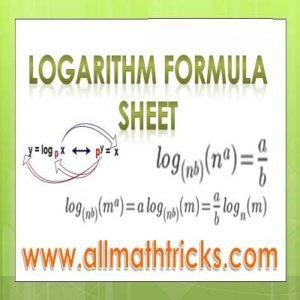# Logarithm Formulas Sheet | Properties of Logarithms | Log Rules | Lows of Log

In this session explained about logarithm formulas ( Laws of Logarithms) like logarithm addition rule and subtract rule and Base change rules and results on logarithmic inequalities with examples

## Log Formula | Logarithm Rules Practice | Logarithm Tutorial | Exercise – 2

Please go through the below link for basic concepts of logarithms like Definition of Logarithm , Characteristic and MantissaRule for write Mantissa and CharacteristicNatural Logarithm , Common Logarithms ..  etc

Logarithm Tutorial | Exercise – 1

### Logarithm formula sheet ( Laws of Logarithms )

#### First Law of the logarithms – ( logarithm addition rule )

” Log of product = Sum of Logs”

log a m n = log a m + log a n ;

Where m, n & a are positive real numbers and a ≠  1

For example –   Take 524 x 254

Let 524 = 10n and 254 = 10m

Now rewrite above exponential forms into logarithmic forms

i.e n = log 10 524  and m = log 10 254

524 x 254 = 10n x 10m

524 x 254 = 10 n+m

Now rewrite above exponential forms into logarithmic forms

n + m  = log 10 (524 x 254)

Substitute ‘n‘ and ‘m‘ vales in the above equation

log 10 524 + log 10 254 = log 10 (524 x 254)

Generalization of the above logarithmic law

log (abc) = log a + log b + log c

log (n1n2n3n4n5 – – – – – – –  nk) = log n1 + log n2 + log n3 + log n4 + log n5   +  – – – – – –  + log nk

(Note : As per log rules, If base is not mentioned for any logarithmic equations  then it is considered as base 10. The logarithmic calculation to base 10 are called common logarithms.)

#### Second Law of the logarithms –  ( logarithm subtract rule )

” log of quotient = difference of logs”

log a (m/n) = log a m  –  log a n ;

Where m, n & a are positive real numbers and a ≠  1

For example –   Take 524 / 254

Let 524 = 10m and 254 = 10n

Now rewrite above exponential forms into logarithmic forms

i.e m = log 10 524  and n = log 10 254

524 /254 = 10m x 10n

524 / 254 = 10 m -n

Now rewrite above exponential forms into logarithmic forms

m – n  = log 10 (524 /254)

Substitute n and ‘m‘ vales in the above equation

log 10 524  –  log 10 254 = log 10 (524/254)

#### Third Law of the logarithms –

log a (m n) = n log a   ;

Where m, n and a are positive real numbers and a ≠  1

For example –   Take 528

Let 52 = 10n    ——  ( 1)

Now rewrite above exponential forms into logarithmic forms

i.e n = log 10 52  —–  ( 2)

Here 52 can be written as 5.2 x 101

Now  528 = (5.2 x 101 )8 = 5.28 x 108

Raising the power to 8 on both sides of the equation ( 1)

528 = (10n )8

⇒ 528 = 108n

Now rewrite above exponential forms into logarithmic forms

8 n   = log 10 (528)

Substituent the value of ‘n’ in the above equation

8 log 10 52  = log 10 (528)

#### Base Change Rules

1.2.  log n m = log a m / log a n   = log a m .  log n a

3.##### Some other Properties of logarithms

3. a log a N = N

4.  log a a = 1

5.6.7.Logarithmic Inequalities

1. If  n > 1 and log n a > log n b then a > b

2. If  n <1 1 and log n a > log n b then a <  b

Please go through the below link for logarithm applications with examples and solutions

Logarithm Tutorial | Exercise – 3Related Articles

Algebra division| Dividing Polynomials Long Division

Factor Theorem Applications| Factor theorem example problems

Remainder Theorem for Number System with Difficult Examples

Lines | Line segments | Rays | All Math Tricks

## 1 thought on “Logarithm Formulas Sheet | Properties of Logarithms | Log Rules | Lows of Log”#### Gopi

(July 22, 2021 - 11:02 am)